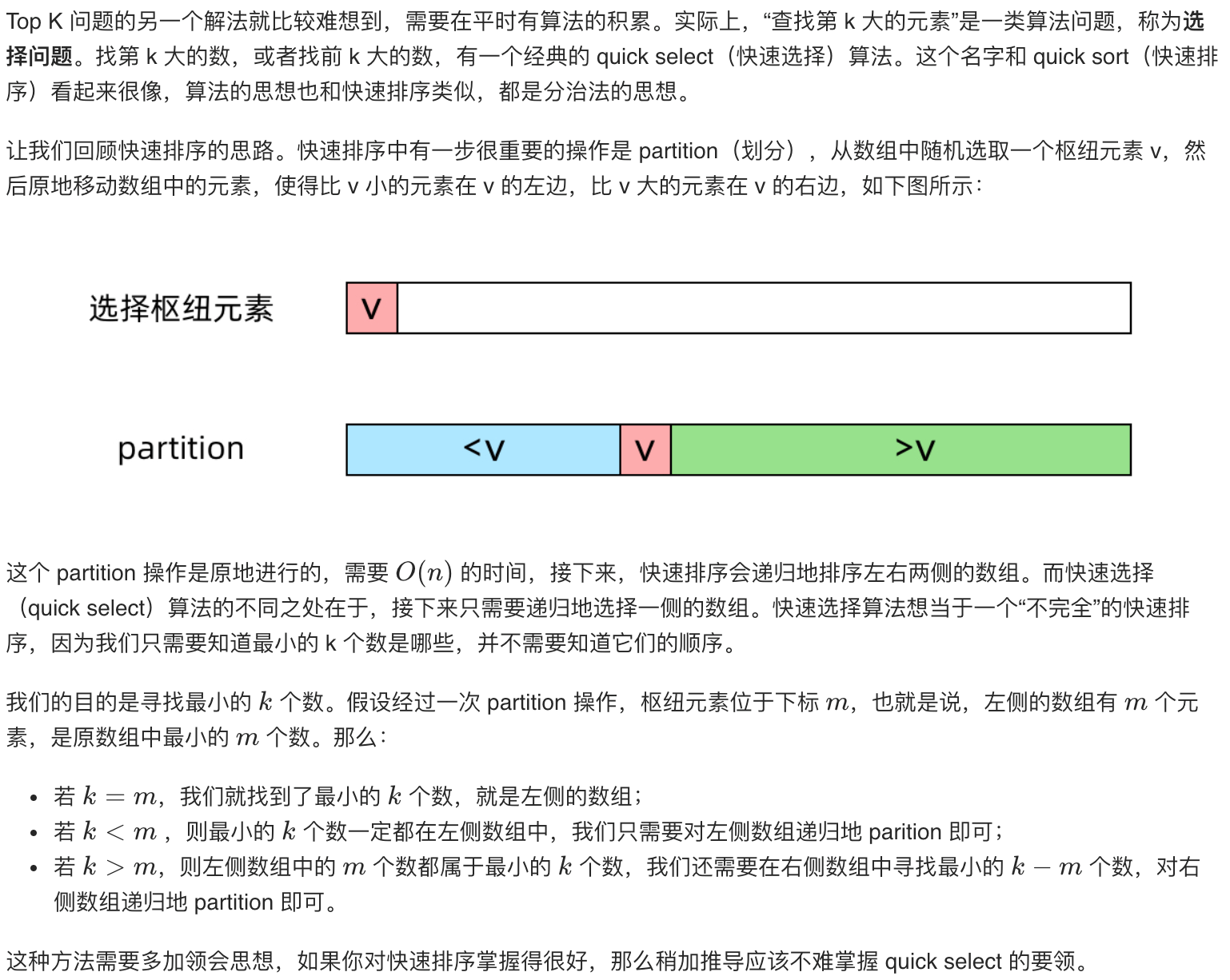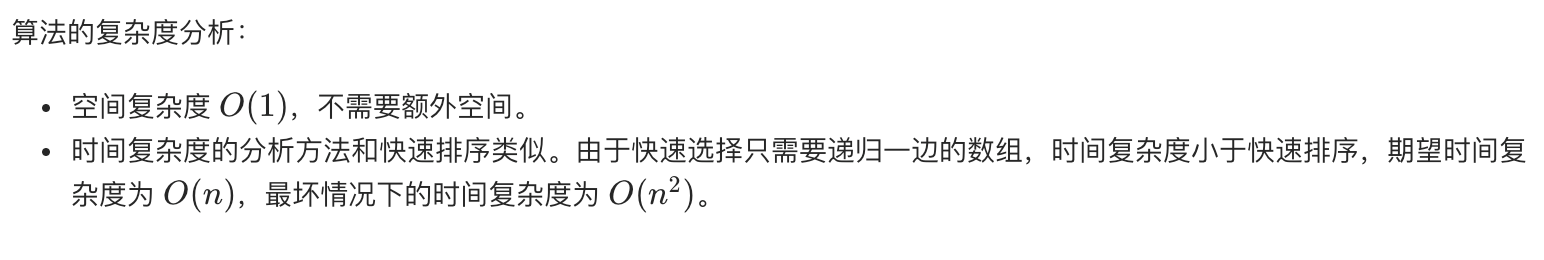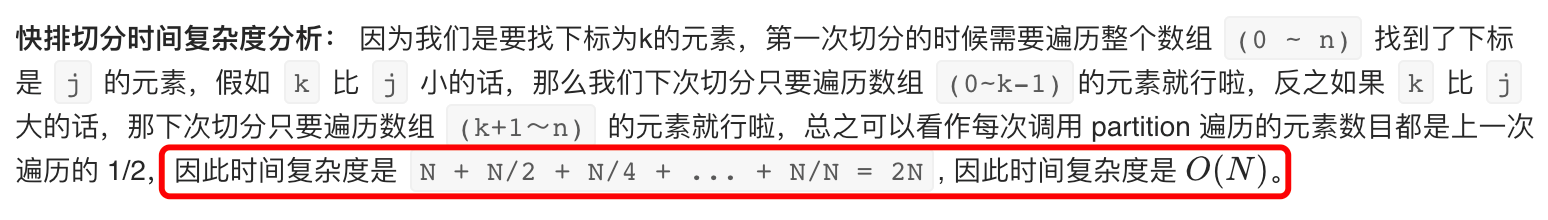# 剑指 Offer 40. 最小的k个数——TopN问题

## 一、题目

• 输入整数数组 arr ，找出其中最小的 k 个数。例如，输入4、5、1、6、2、7、3、8这8个数字，则最小的4个数字是1、2、3、4。

• 示例 1：
输入：arr = [3,2,1], k = 2
输出：[1,2] 或者 [2,1]

• 示例 2：
输入：arr = [0,1,2,1], k = 1
输出：

• 限制：
0 <= k <= arr.length <= 10000
0 <= arr[i] <= 10000

• 来源：力扣（LeetCode）
链接：剑指 Offer 40. 最小的k个数

https://blog.csdn.net/lightupworld/article/details/106684684

## 二、题解分析

• 注意找前 K 大/前 K 小问题不需要对整个数组进行 O(NlogN)的排序！
例如本题，直接通过快排切分排好第 K 小的数（下标为 K-1），那么它左边的数就是比它小的另外 K-1 个数啦～

• 我们可以借鉴快速排序的思想。我们知道快排的划分函数每次执行完后都能将数组分成两个部分，小于等于分界值 pivot 的元素的都会被放到数组的左边，大于的都会被放到数组的右边，然后返回分界值的下标。与快速排序不同的是，快速排序会根据分界值的下标递归处理划分的两侧，而这里我们只处理划分的一边

•## 三、题解

class Solution {
public int[] getLeastNumbers(int[] arr, int k) {

//0.异常处理
if(k == 0 || arr.length == 0 ) return new int;
if(arr.length <= k) return arr;

//寻找第K大的元素
quickSearch(arr,0,arr.length-1,k);

//返回前k大数

int[] res = new int[k];

for(int i =0; i<k; i++){
res[i] = arr[i];
}
return res;
}

//定义快速搜索第K个元素

private void quickSearch(int[] arr, int low, int hight, int k){

int p = quickPartition(arr,low,hight);

//判断p 和 K 的关系。

if( p == k){
//如果相等 p左边元素 就是结果
return; //arr - arr[p-1]就是结果
}else if( p < k){ //第k小的数在 p的的右侧，需要递归调用 quickSearch
quickSearch(arr,p+1,hight,k);

}else{  // p > k  第K小的数 在 P的左侧

quickSearch(arr,low,p-1,k);
}
}

//定义快排函数 不要递归部分 主要是分区部分 返回分区点

private int quickPartition(int[] arr, int low, int hight){

//1.定义递归结束条件

if(low > hight) return -1;

//2.定义哨兵 和基点
int i = low, j= hight, temp = arr[low];

//3.循环遍历分区
while(i<j){

while(i<j && arr[j]>=temp) j--; // >=
while(i<j && arr[i]<=temp) i++; // <=

//交换arr[i] arr[j]
if(i < j){
int tmp = arr[i];
arr[i] = arr[j];
arr[j] = tmp;
}
}
//4.交换基点
arr[low] = arr[i];
arr[i] = temp;

//5递归左右， 这里不需要
//6.本题额外代码 返回一次分区后的界点

return j ; //j左边[0,j-1] 的数 都小于 arr[j]
}
}


## 四、复杂度分析• 注 快排最好时间 O(n) 平均 O(nlogn) 最坏 O(n^2) 空间O(logN) (递归调用占用)
• 本例中的时间复杂度 每次切分一半， n -> n/2 和快排最好的时间复杂度是一种情况 所以也是O(n)
08-0223005-265176
06-02646
02-052878
11-0152
07-10478
12-271446
12-271471
11-221587
03-022125